Related Articles
Activation functions in Neural Networks | Set2
• Last Updated : 05 Sep, 2020

The article Activation-functions-neural-networks will help to understand the use of activation function along with the explanation of some of its variants like linear, sigmoid, tanh, Relu and softmax. There are some other variants of the activation function like Elu, Selu, Leaky Relu, Softsign and Softplus which are discussed briefly in this article.

Leaky Relu function:
Leaky Rectified linear unit(Leaky Relu) is an extension of the Relu function to overcome the dying neuron problem.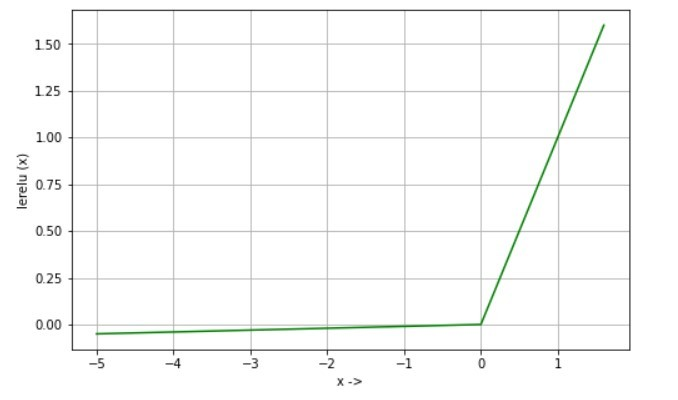Equation:

```lerelu(x) =  x if x>0
lerelu(x) = 0.01 * x if x<=0 ```

Derivative:

```d/dx lerelu(x) =  1 if x>0
d/dx lerelu(x) = 0.01 if x<=0
```

Uses: Relu return 0 if the input is negative and hence the neuron becomes inactive as it does not contribute to gradient flow. Leaky Relu overcomes this problem by allowing small value to flow when the input is negative. So, if the learning is too slow using Relu, one can try using Leaky Relu to see any improvement happens or not.

Elu function:
The exponential Linear Unit is also similar to Leaky Relu but differs for negative input. It also helps to overcome the dying neuron problem.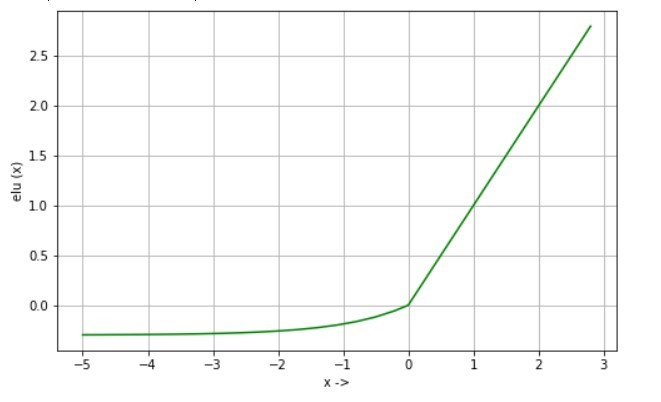Equation:

```elu(x) =  x if x>0
elu(x) = alpha * (exp(x)-1) if x<0
```

Derivative:

```d/dx elu(x) =  1 if x>0
d/dx elu(x) = elu(x) + alpha if x<=0
```

Uses: It has the same purpose that of Leaky Relu and convergence of cost function towards zero is faster than Relu as well as Leaky Relu. For example, neural network learning on Imagenet using Elu is faster than using Relu.

Selu function:
Scaled Exponential Linear Unit is the scaled form of Elu. Just multiply the output of Elu by a predetermined “scale” parameter and you will get the desired output which selu gives.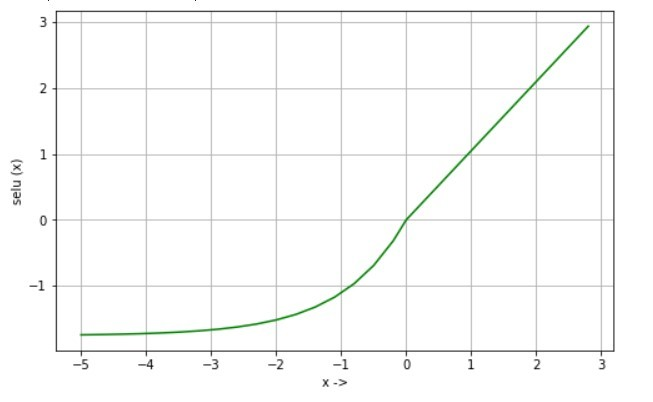Equation:

```selu(x) =  scale * x if x>0
selu(x) = scale * alpha * (exp(x)-1) if x<=0
where,
alpha = 1.67326324
scale = 1.05070098
```

Derivative:

```d/dx selu(x) = scale if x>0
d/dx selu(x) = selu(x) + scale * alpha if x<=0
```

Uses: This activation function is used in Self-Normalizing Neural Networks (SNNs) which is used to train a deep and robust network less effected from vanishing and exploding gradient problem.

Softsign function:
Softsign function is an alternative to tanh function where tanh converges exponentially and softsign converges polynomially.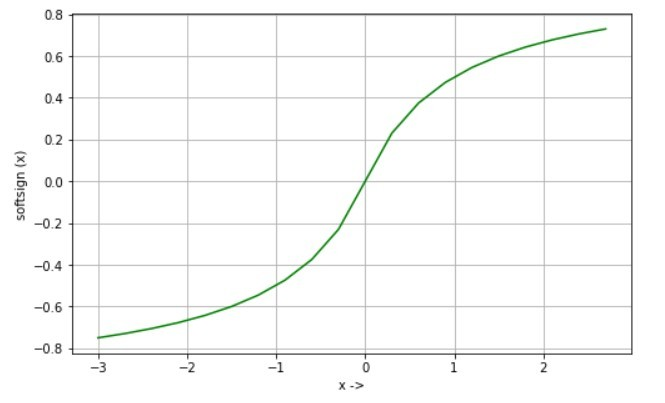Equation:

```softsign(x) = x / (1 + |x|)
```

Derivative:

```d/dx softsign(x) = 1 / (1 + |x|)^2
```

Uses: It is mostly used in the regression problem and can be used in a deep neural network for text to speech conversion.

Softplus function:
Softplus function is a smoothed form of the Relu activation function and its derivative is the sigmoid function. It also helps in overcoming the dying neuron problem.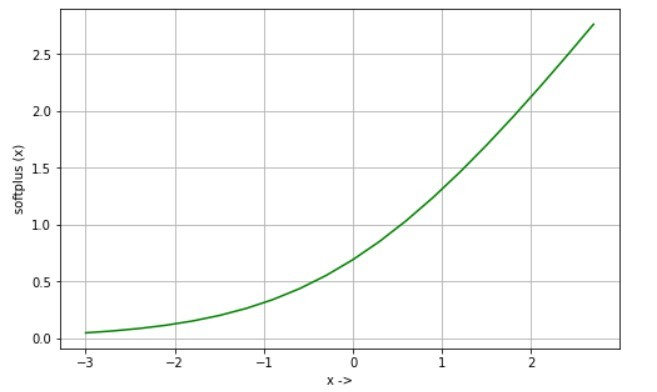Equation:

```softplus(x) = log(1 + exp(x))
```

Derivative:

```d/dx softplus(x) = 1 / (1 + exp(-x))
```

Uses: Some experiments show that softplus takes lesser epochs to converge than Relu and sigmoid. It can be used in the speech recognition system.My Personal Notes arrow_drop_up